AP Calculus AB : Integrals

Example Questions

Example Question #61 : Integrals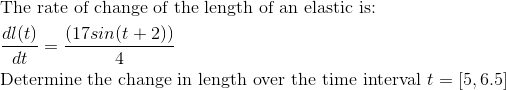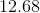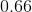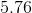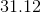Explanation: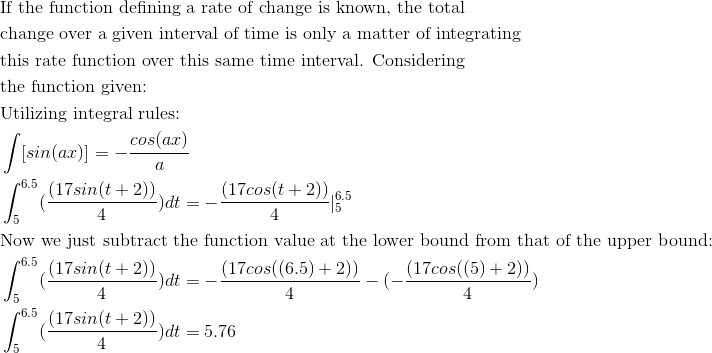Example Question #62 : Integrals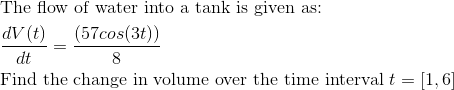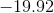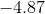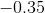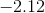Explanation: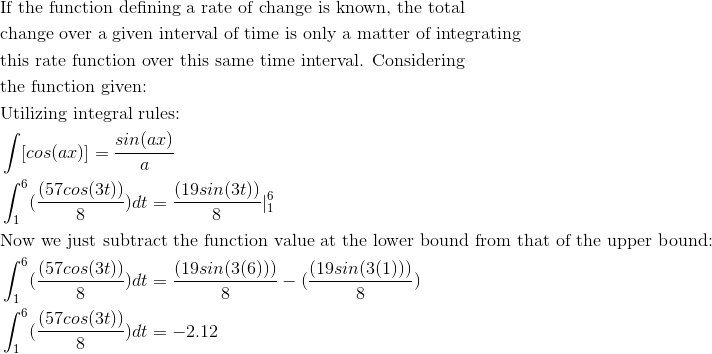Example Question #63 : Integrals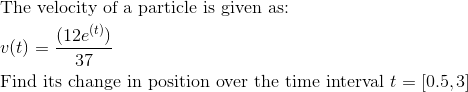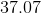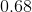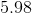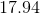Explanation: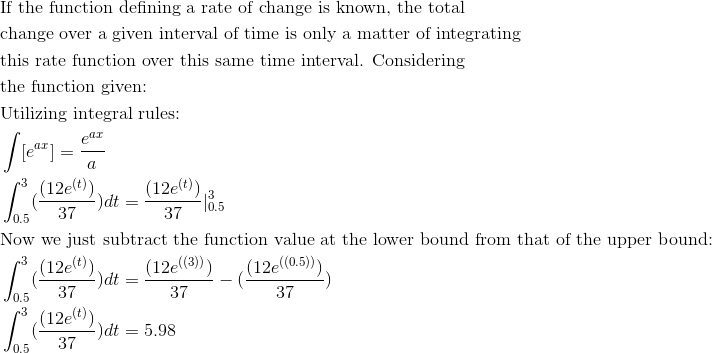Example Question #64 : Integrals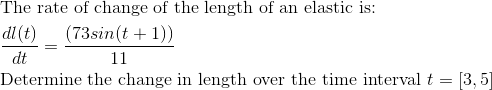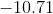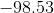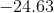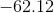Explanation: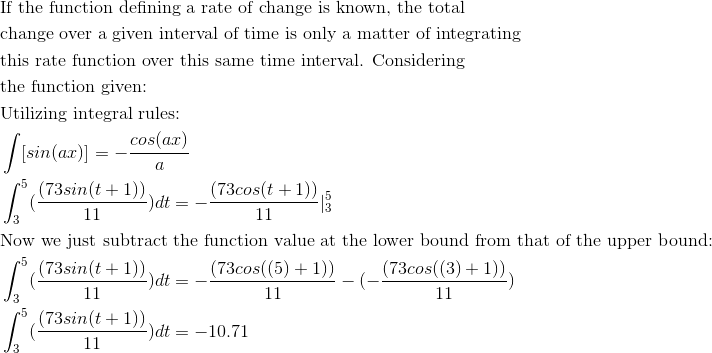Example Question #65 : Integrals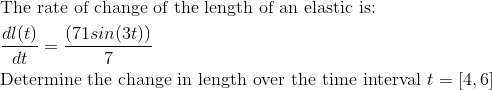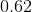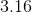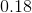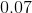Explanation: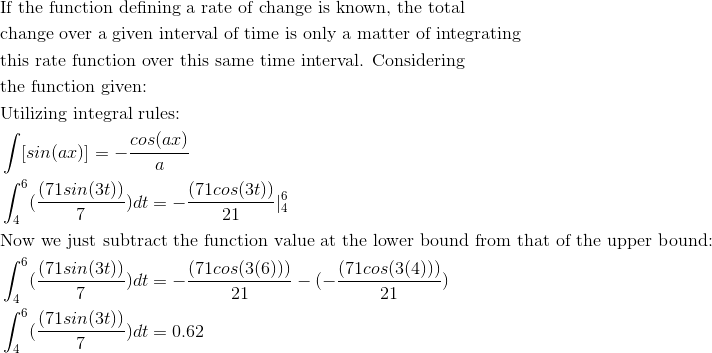Example Question #66 : Integrals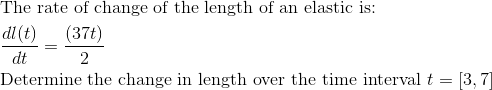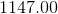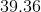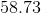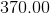Explanation: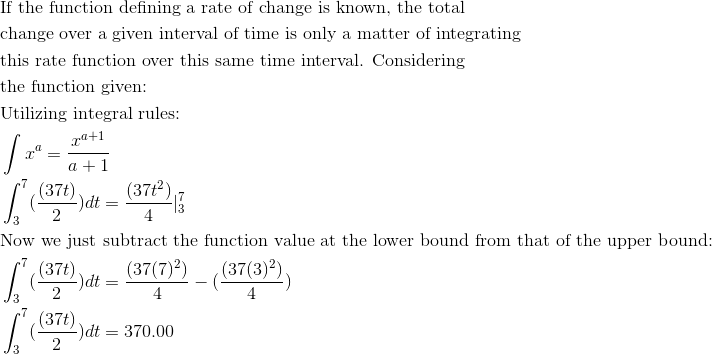Example Question #67 : Integrals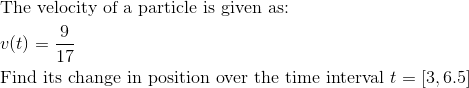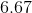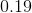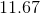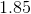Explanation: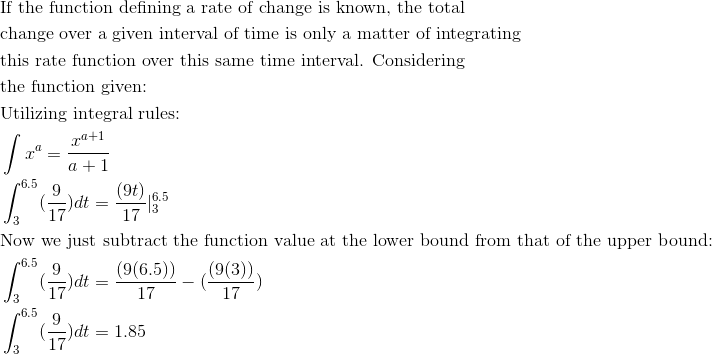Example Question #68 : Integrals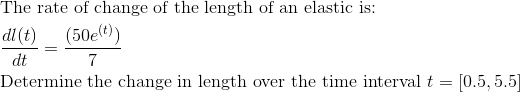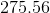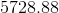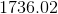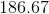Explanation: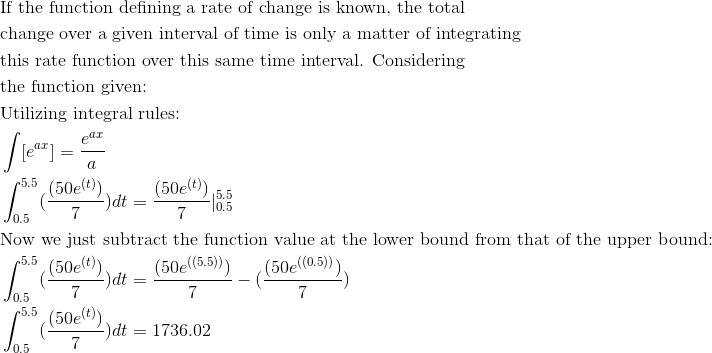Example Question #21 : Definite Integral Of The Rate Of Change Of A Quantity Over An Interval Interpreted As The Change Of The Quantity Over The Interval

A pot of water begins at a temperature of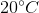and is heated at a rate of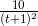degrees Celsius per minute. What will the temperature of the water be after 4 minutes?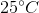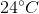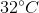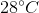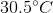Explanation:

Let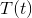denote the temperature of the pot afterminutes.

The first thing to realize is that the quantity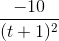is the derivative of. Using the fundamental theorem of Calculus, we know that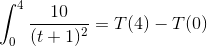From there, we just need to solve the integral. Letting u = t+1, du=dt, we have the following: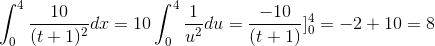Where the second equality follows by the power rule, and re-substituting t+1 = u.

Thus, we now have the equation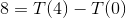. Because we know that the water started at, all we need to do is rearrange and substitute.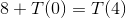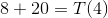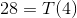Yielding our final answer,Example Question #69 : Integrals

A ball is thrown into the air. It's height, after t seconds is modeled by the formula:

h(t)=-15t^2+30t feet.

At what time will the velocity equal zero?

0s

3s

5s

1.5s

1s

1s

Explanation:

In order to find where the velocity is equal to zero, take the derivative of the function and set it equal to zero.

h(t) = –15t+ 30t

h'(t) = –30t + 30

0 = –30t + 30

Then solve for "t".

–30 = –30t

t = 1

The velocity will be 0 at 1 second.